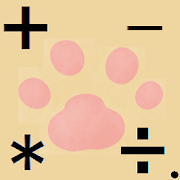# CatPaw Calc SimpleEveryone
The calculator has following functions.
(1)Four arithmetic and power operations(Simple mode)
(2)Expressions and the calculation results are recorded as History.
(3)Calculation of value-added tax(Calculation of value included tax from value without tax)
(4)Value of (3) can be recorded ,added to the memory.
(5)Value of "input number/calculating result textview" can be recorded ,added to,subtracted from the memory(that is different from memory of (4)).
(6)The computation for scientific function ( Advance mode )

1.How to calculate
The screen has textviews for displaying input expression ,input numbers or calculation result and tax calculation value above keyboard for input(for numbers and operators ).
(example)
123-10/5 = <--input expression
121 0 <--input numbers/calculation result(121) tax calculation value(0)

To calculate expressions, you input numbers and operators(+,-,*,/,^) with keyboard on the screen.
* is multiplication , / is division and ^ is power operation(for example 2^3 is 3rd power of 2 and the result is 8). You can use a decimal point for the exponent numbers(numbers after ^).
However "Simple mode" doesn't use √ key ,you can calculate square root using ^ key. For example when you find the square root of 2 ,enter 2 ,^ ,( ,1 ,/ ,2 and put ) key at the end ,that is 2^(1/2) equals the square root of 2.
(In "Advance mode",you can use √ Key.)
Putting C key clears all contents of textviews for expressions ,input numerical values or calculation results and tax calculation value.
When you tap CE key ,the textview for input numerical values or calculation results are cleared.
The DEL key undo the last operation.
The tax key is used for calculating value-added tax or consumption tax.
When you tap the tax key ,the value in input number/calculating result textview is multiplied by setting tax rate and the result is shown in tax calculation textview.
Tax rate and rounding methods is set by [Set tax calculation]dialog opened by [Set tax calculation]menu item in action menu(...) on top right of the screen.
If you do a long press of numbers on tax calculation textview ,the context menu is opened. By selecting items on this menu ,you can add tax calculation value to the memory and retrieve current memory value and other memory operations.

When the keyboard protrudes from the screen because of too small display ,swipe the screen from right to left.

2.The explanation of menu on Calculation screen(that is shown after starting of app)
This menu has 5 items and the function is as follows.

a)Set tax calculation...[Set tax calculation]dialog is shown. By using the dialog ,you can set tax rate and rounding method for tax calculation.
b)Set showing tax rate dialog on startup...[Set tax calculation]dialog is automatically called after first starting the app ,but at startup of second time or after the dialog is not shown. However this item is tapped ,at the next startup the dialog is opened.
C)Showing history...History(list of expressions and the answers) screen is opened.
d)outp:irreducible fraction/floating-point number...Select format of calculation result
e)Change calc key pad...Displaying the dialog that you can switch "Simple" mode and "Advance" mode.

3.Explanation of rounding methods in tax calculation
When you tap the Tax key ,the calculation result is rounded to the nearest integer.You can select the rounding method by using [Set tax calculation]dialog.
The detail is described in http://www.amigo2.ne.jp/~s_mouse/sub/appe/catpawcalc/howtouse.html.

4.Explanation of Memory Key( Advance mode)
The memory function for input/calculation result is available in Advance mode.Visit the Help page on details ,please.

5.Scientific function
Calculation for function such as loglithum ,exponential, trigonometric functions is available in Advance mode.
Collapse

Review Policy

## What's New

Scientific functions such as trigonometric function have been appended to "Advance mode".
"Help" item is added to the Menu.Using the item starts browser and opens help page.
Following bugs are fixed.
a)At after the second decimal point,if you try to input 0 after 0 ,the second 0 is not shown.
b)After inputting a formula with %, the application crashes when you open History page or restart the app.
Collapse

Updated
May 9, 2018
Size
2.7M
Installs
100+
Current Version
4.0
Requires Android
4.0.3 and up
Content Rating
Everyone
Permissions
Offered By
StellarMouse